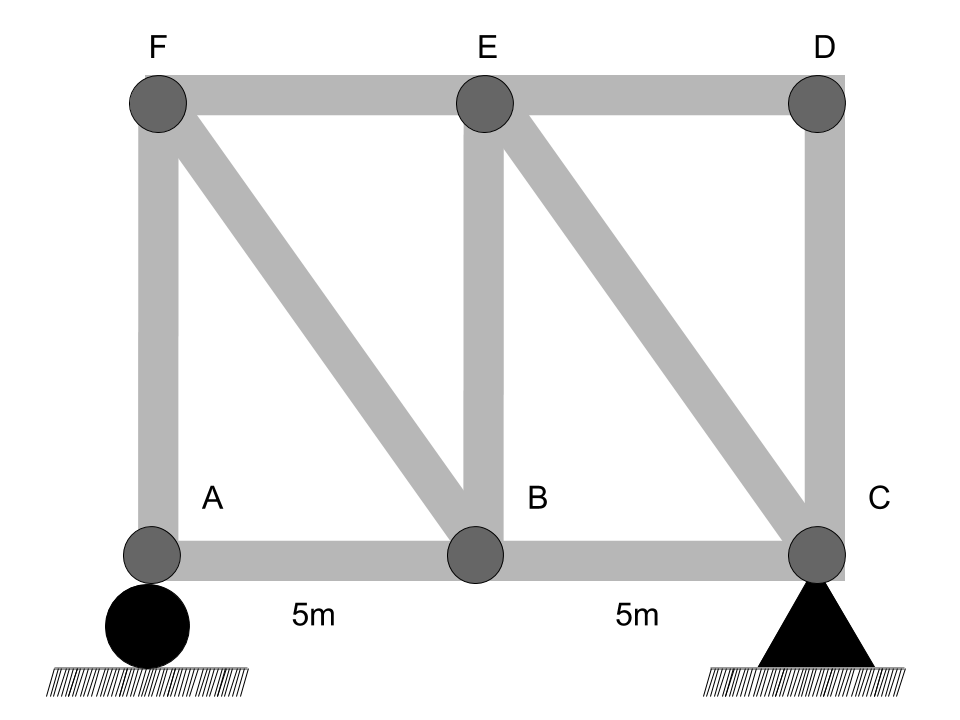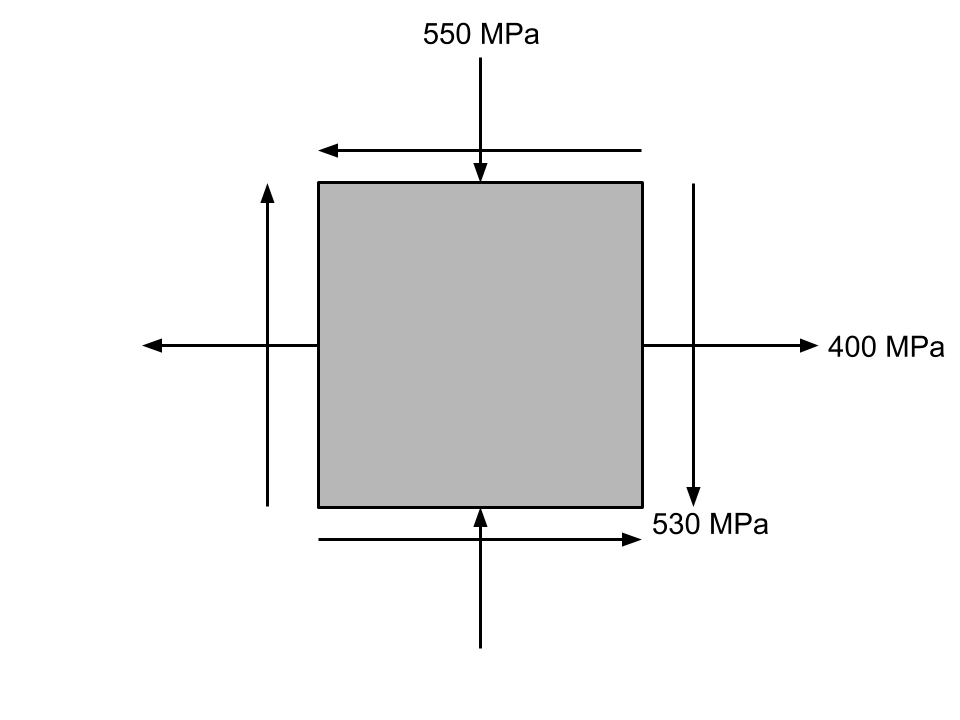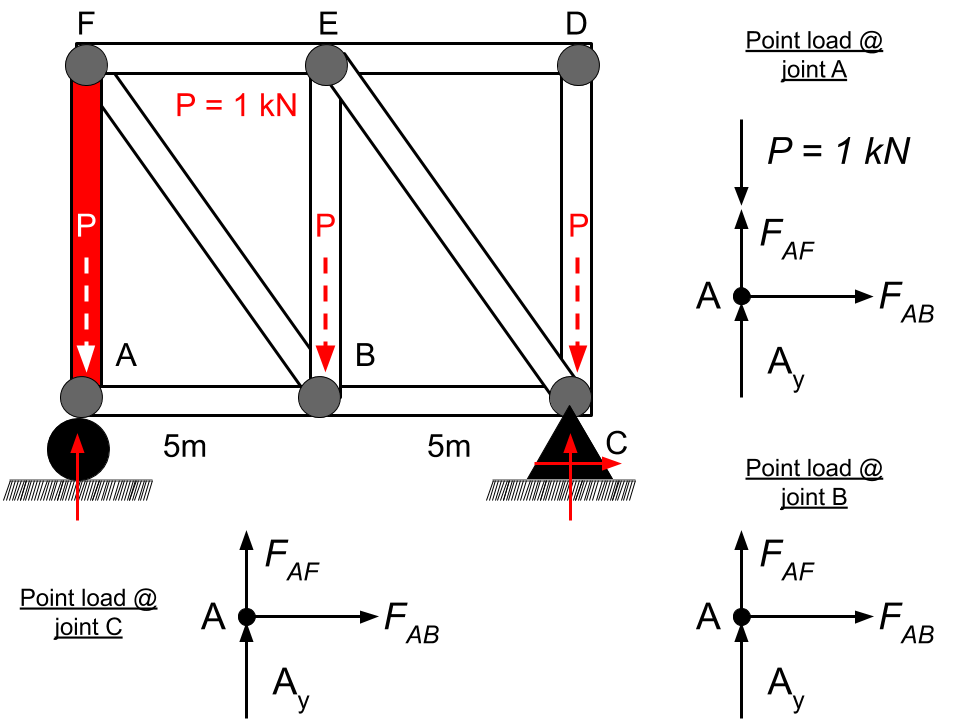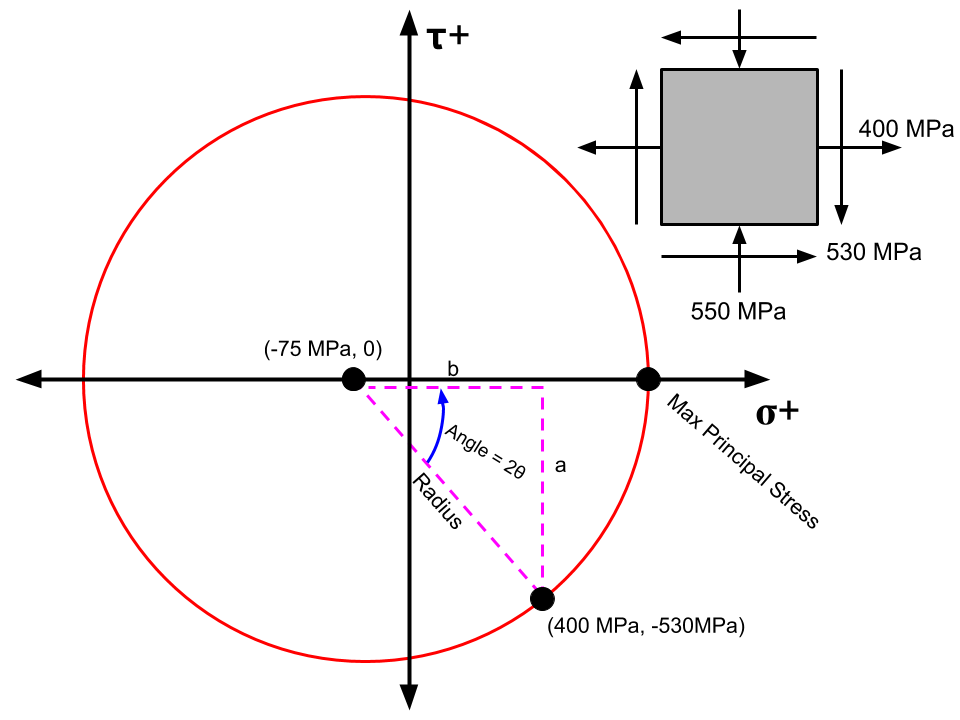Updated for the new July 2020 FE Exam Topics

Free FE Practice Test

# PrepFE™

## Free FE Civil Example Practice Problems

We've selected 10 diverse practice problems from our question bank that you can use to review for the Civil engineering FE exam and give you an idea about some of the content we provide.

### 1) A table has been set up to aid in the drawing of an influence line. What is most nearly the internal force in member AF when the influence line point load is placed at joint B? Assume the moving point load is equal to 1kN. $$\begin{array}{c|c} \text{Point Load Location} & F_{AF} \\ \hline \text{Joint A} & 0\si{kN} \\ \text{Joint B} & - \\ \text{Joint C} & 0\si{kN} \\ \end{array}$$### 2) What is most nearly the bending moment in the beam when shear equals zero?### 3) What is the angle of rotation needed to get the stress element in the maximum principal stress state?### 9) What is most nearly the area moment of inertia about the x-axis of the irregular shape shown below?## Solutions

### 1) A table has been set up to aid in the drawing of an influence line. What is most nearly the internal force in member AF when the influence line point load is placed at joint B? Assume the moving point load is equal to 1kN. $$\begin{array}{c|c} \text{Point Load Location} & F_{AF} \\ \hline \text{Joint A} & 0\si{kN} \\ \text{Joint B} & - \\ \text{Joint C} & 0\si{kN} \\ \end{array}$$A.2.5 kN
B.1 kN
C.0 kN
D.0.5 kN

### Explanation:An influence line shows the effect a point load has on a truss member as the point load moves along the truss. In other words, what's the internal force in a truss member when the point load is at joint A, joint B, joint C, etc.

We can tabulate the internal force of member AF as the point load goes from joint A to joint C. $$\begin{array}{c|c} \text{Point Load Location} & F_{AF} \\ \hline \text{Joint A} & 0\si{kN} \\ \text{Joint B} & 0.5\si{kN} \\ \text{Joint C} & 0\si{kN} \\ \end{array}$$ When point load is at joint A $$\curvearrowleft +\sum M_C=-A_y(5+5\si{m})+1\si{kN}(5+5\si{m})=0 \\ \therefore A_y=1\si{kN}$$ Method of joints at joint A:
$$\sum F_y=F_{AF}-P+A_y=0 \\ \sum F_y=F_{AF}-1\si{kN}+1\si{kN}=0 \\ \therefore F_{AF}=0$$ When point load is at joint B $$\curvearrowleft +\sum M_C=-A_y(5+5\si{m})+1\si{kN}(5\si{m})=0 \\ \therefore A_y=0.5\si{kN}$$ Method of joints at joint A:
$$\sum F_y=F_{AF}+A_y=0 \\ \sum F_y=F_{AF}+0.5\si{kN}=0 \\ \therefore F_{AF}=-0.5\si{kN}$$ When point load is at joint C $$\curvearrowleft +\sum M_C=-A_y(5+5\si{m})=0 \\ \therefore A_y=0\si{kN}$$ Method of joints at joint A:
$$\sum F_y=F_{AF}+A_y=0 \\ \sum F_y=F_{AF}+0\si{kN}=0 \\ \therefore F_{AF}=0\si{kN}$$

### 2) What is most nearly the bending moment in the beam when shear equals zero?A.4.21 kN-m
B.1.69 kN-m
C.3.88 kN-m
D.11.22 kN-m

### Explanation:The FE Reference Handbook provides equations that you can use to quickly calculate the maximum moment or shear for certain beam loading-support conditions. Search for simply supported beam on the FE Reference Handbook to find a table that has max shear and max moment equations for different types of beams. In this particular example, this beam loading-support condition is not part of the table.

Therefore, use shear and moment diagrams to determine the maximum bending moment in the beam.

Steps to draw shear and moment diagrams:
1. Draw a free body diagram of the beam.
2. Determine all the reactions and moments by using the equilibrium equations.
3. Draw the shear and moment diagrams by slicing the beam into sections.

Step 1: Draw a FBD.
Roller supports will have a vertical reaction force. Pin supports will have a vertical and a horizontal reaction force.

Step 2: Use equilibrium equations to solve for all the forces on the FBD. $$\sum M=\text{Force}\cdot\text{Distance}=0 \\ \sum F_x=0, \sum F_y=0$$
$$\sum M_A=\left[(-6\si{kN/m})(2\si{m}+1\si{m})\right](\frac{2\si{m}+1\si{m}}{2})+B_y(2\si{m})=0 \\ B_y=13.5\si{kN}$$ Side note: we divide the distance arm (2m+1m) by 2 to get the resultant for the moment generated by the 6kN/m load. $$\sum F_y=\left[(-6\si{kN/m})(2\si{m}+1\si{m})\right]+13.5\si{kN}+A_y=0 \\ A_y=4.5\si{kN}$$ $$\sum F_x=A_x=0 \\A_x=0$$

Step 3: Develop the shear and moment diagrams by slicing the beam into sections.

Looking at slice #1. Develop a shear equation when $0\leq x \leq2\si{m}$ $$\sum F_y = 4.5\si{kN}-(6\si{kN/m}\cdot x)-v=0 \\ v=4.5\si{kN}-(6\si{kN/m}\cdot x)$$ Looking at slice #1. Develop a moment equation when $0\lt x \leq2\si{m}$ $$\sum M_{\text{right side}} = \left[(+6\si{kN/m})(x)\right](\frac{x}{2})-4.5\si{kN}(x)+M=0 \\ M=(+4.5\si{kN})(x)-(6\si{kN/m}\cdot x)(\frac{x}{2})$$ Looking at slice #2. Develop a shear equation when $2\lt x \leq 3\si{m}$ $$\sum F_y = 4.5\si{kN}-(6\si{kN/m}\cdot x)-v+13.5\si{kN}=0 \\ v=18\si{kN}-(6\si{kN/m}\cdot x)$$ Looking at slice #2. Develop a moment equation when $2\lt x \leq 3\si{m}$ $$\sum M_{A} = \left[(-6\si{kN/m})(x)\right](\frac{x}{2})+13.5\si{kN}(2\si{m}) \\ +M-\left[18\si{kN}-(6\si{kN/m}\cdot x)\right](x)=0$$
$$M=3x^2-27+18x-6x^2$$ Now that we have the shear and moment equations for different sections of the beam, we can use these equations to plot the shear and moment diagrams.

$$\begin{array}{c|c|c} \text{x} & \text{Shear (v)}& \text{Moment (M)} \\ \hline 0\si{m} & 4.5\si{kN} & 0\si{kNm} \\ 1\si{m} & -1.5\si{kN} & 1.5\si{kNm} \\ 1.99\si{m} & -7.44\si{kN} & -2.92\si{kNm} \\ 2.10\si{m} & 5.40\si{kN} & -2.43\si{kNm} \\ 3\si{m} & 0\si{kN} & 0\si{kNm} \\ \end{array}$$ We know the maximum moment occurs when shear is equal to zero. And based on the table above, we know zero shear occurs somewhere where $0\leq x \leq 2\si{m}$. Therefore, if we set shear equal to zero and use the shear equation when $0\leq x \leq 2\si{m}$ then we can find where along the beam shear=0 occurs. And then if we know the x value, then we use the moment equation to determine the moment for the given x.

Looking at slice #1 $0\leq x \leq2\si{m}$ $$\sum F_y = 4.5\si{kN}-(6\si{kN/m}\cdot x)-v=0 \\ \sum F_y = 4.5\si{kN}-(6\si{kN/m}\cdot x)-(0)=0 \\ x=0.75\si{m} \text{ when shear = 0}$$ Max moment occurs when shear is zero, therefore $$M=(+4.5\si{kN})(x)-(6\si{kN/m}\cdot x)(\frac{x}{2}) \\ M=(+4.5\si{kN})(0.75)-(6\si{kN/m}\cdot 0.75)(\frac{0.75}{2}) \\ M_{max}=1.69\si{kNm}$$

Side note: you're unlikely to encounter a problem in the FE that requires these many steps. However, it's safe to assume that you could encounter a problem where different portions of this question are asked as separate problems. If you fully understand this problem, you should be ready for any shear-moment diagram problem you could face during the actual FE.

### 3) What is the angle of rotation needed to get the stress element in the maximum principal stress state?A.$10^\circ$CCW
B.$36^\circ$CCW
C.$48^\circ$CCW
D.$24^\circ$CCW

### Explanation:Visit the Mohr's circle section in the Mechanics of Materials chapter of the FE Reference Handbook.

Ultimately, we must determine the angle rotation needed to get the stress element to a principal stress state.

Steps to determine the angle of rotation needed:
1. Draw a quick Mohr's circle based on what's shown in the stress element.
2. Draw a right triangle from the center of the Mohr's circle to a known point along the circle. Determine the Mohr's circle's angle between the known point and the sigma axis (x-axis), which is where the principal stress occurs. That will be the Mohr's circle rotation angle needed to get to a principal stress state.
3. Convert the Mohr's circle's rotation angle needed to get to a principal stress state to the stress element's angle needed to get to a principal stress state.

Step 1. Draw a quick Mohr's circle based on what's shown in the stress element.

Refer to the stress element shown in the FE Reference Handbook to understand its sign notation. Normal stresses ($\sigma_x,\sigma_y)$ in tension (going away from the stress element) are positive and normal stresses in compression (going into the stress element) are negative. For shear stresses ($\tau_{xy}$), look at the right side of the stress element. If the right shear arrow pulls the stress element clockwise, then the shear is negative. If the right shear arrow pulls the stress element counter clockwise, then the shear is positive. Therefore,

$\tau_{xy}=-530 \si{MPa} \\ \sigma_{x}=400\si{MPa} \\ \sigma_{y}=-550 \si{MPa}$

Determine the center of the Mohr's circle. $$C=\frac{\sigma_x+\sigma_y}{2}=\frac{400+(-550)}{2}=-75\si{MPa}$$ With the known info, plot a random point along the perimeter of the circle.

$$(\sigma_x,\tau_{xy})=(400,-530)$$

Step 2. Plot a right triangle from the center of the Mohr's circle to the known point along the perimeter of the circle.

Use Pythagoras Theorem to solve for the hypotenuse of the triangle, which is also the radius of the circle. $$a^2+b^2=hypotenuse^2 \\ (530)^2+(75+400)^2=hypotenuse^2 \\ hypotenuse=radius=711.7\si{MPa}$$ The angle, 2$\theta$, is the Mohr's circle's angle to get to a principal stress state. The rotation angle needed to get the stress element to a principal stress state is $\theta$. This problem asks for the angle to get the stress element to a principal stress state.

Calculate the Mohr's circle's angle to a principal stress state. Use soh-cah-toa. $$\sin{\left[\frac{opposite}{hypotenuse}\right]}^{-1}=angle \\ \sin{\left[\frac{530}{711.6}\right]}^{-1}=48^\circ$$ Step 3. Convert the Mohr's circle's rotation angle needed to get to a principal stress state to the stress element's angle needed to get to a principal stress state.

$$\text{Mohr's Circle's angle}=2\theta=48^\circ$$ Stress element's rotation angle to a principal stress state$\theta=24^\circ$ counterclockwise

A.452.41 ft
B.457.32 ft
C.458.33 ft
D.456.13 ft

### Explanation:HI = Elevation of the surveying instrument from datum
Elev. = Station elevation from datum

Ultimately, we must solve for the elevation at station 2+00. By looking at the figure, we can infer that $$\text{Elevation}_\text{2+00}=\text{HI}_\text{1+00 to 2+00}-\text{FS}_\text{2+00}$$ First find $\text{HI}_\text{1+00 to 2+00}$. By looking at the figure, we can infer that $$\text{HI}_\text{1+00 to 2+00}=\text{Elev}_\text{1+00}+\text{BS}_\text{1+00}$$ $$\text{HI}_\text{1+00 to 2+00}=450.21'+8.12'=458.33'$$ Now go back and solve for the elevation at station 2+00 $$\text{Elevation}_\text{2+00}=458.33'-5.92'=452.41'$$

During the actual FE, you will have to quickly sketch out the figure above in order to come up with a solution. Just remember what HI, BS, and FS mean and you'll be prepared for this question.

A.0.3 in
B.0.1 in
C.0.9 in
D.1.2 in

### Explanation:

Refer to the Geotechnical section in the Civil Engineering chapter of the FE Reference Handbook.

Determine $p_0$, $\Delta p$, and $p_c$ to figure out which consolidation/settlement equation to use. There are three possible equations for consolidation/settlement. We must choose the right one.

$p_0$ is the initial effective consolidation stress, $\sigma_0'$, i.e. the total stress at the midpoint of the layer in question minus the pore water pressure. $$p_o=\sigma_0'=\sigma_0-u$$ where $\sigma_0$ is the total stress at the mid point of the layer in question. In this case, the stress at the mid point of the clay layer will be the sum of the stresses above the mid point. $$\sigma_0=\sum(H \cdot \gamma)$$ $$=\sigma_{\text{fill layer}}+\sigma_{\text{half of clay layer}}$$ $$=\left[ (10')(121\si{pcf})+(\frac{7}{2}')(119\si{pcf}) \right]$$ $$\sigma_0=1,626.5\si{psf}$$ Since we were not told about a ground water table, we will assume the ground is dry and the water table is somewhere below our layers in question. Therefore, porewater pressure, $u$, is zero.

Now go back and solve for $p_0$ $$p_0=1,626.5-0=1,626.5\si{psf}$$ We were given $\Delta p$ to be equal to 100 psf.

We were told in the problem statement this was a normally consolidated clay layer, therefore $p_c=0$.

Now, figure out the appropriate settlement equation to use. $$p_0\geq p_c \text{ and }\Delta p\geq p_c$$ $$1,626.5\si{psf}\geq 0\si{psf} \text{ and }100\si{psf}\geq 0\si{psf}\text{ ✔}$$ Thus $$\Delta H=\frac{H_0}{1+e_0}\left[C_c\log(\frac{p_0+\Delta p}{p_0})\right]$$ $$\Delta H=\frac{7'}{1+0.85}\left[0.252\log(\frac{1,626.5+100}{1,626.5})\right]$$ $$\Delta H =0.0247\si{ft}=0.3\si{in}$$

A.3.2 in
B.1.7 in
C.1.9 in
D.2.4 in

### Explanation:

Refer to the Hydrology/Water Resources section in the Civil Engineering chapter of the FE Reference Handbook.

Ultimately, we must solve for runoff. $$\text{Runoff}=Q=\frac{(P-0.2S)^2}{P+0.8S}$$ Calculate the unknowns $S$ and $P$. $$S=\frac{1000}{CN}-10$$ $$S=\frac{1000}{97}-10=0.3093\si{in}$$ $$\text{Precipitation}=P=2.75\si{in}$$ Calculate the amount of runoff, $Q$. $$Q=\text{Runoff}=\frac{\left[2.75\text{"}-0.2(0.3093\text{"})\right]^2}{2.75\text{"}+0.8(0.3093\text{"})}$$ $$Q=\text{Runoff}=2.4\si{in}$$

A.93 ft
B.4,550 ft
C.1,023 ft
D.950 ft

### Explanation:

Refer to Vertical Curves: Sight Distance Related to Curve Length table - Transportation section in the Civil Engineering chapter of the FE Reference Handbook.

We must determine, $L$, when $S\leq L$ and when $S\gt L$

Side Note: The FE Reference Handbook refers to stopping sight distance as S and also as SSD. Sometimes this causes confusion. Keep in mind.

When $S\gt L$, sag vertical curves - headlight criteria $$L=2(S) - \left[ \frac{400+3.5(S)}{A} \right]$$ $$A=\lvert \text{grade difference}\rvert= \lvert 2.5 -(-3)\rvert= 5.5$$ $$L=2(750\si{ft}) - \left[ \frac{400+3.5(750\si{ft})}{5.5} \right]=950'$$ Check if $S\gt L \Rightarrow 750' \lt 950'$. Condition not true. Reject.

When $S\leq L$, sag vertical curves - headlight criteria $$L=\frac{AS^2}{400+3.5(S)}$$ $$L=\frac{(5.5)(750\si{ft})^2}{400+3.5(750\si{ft})}=1,022.73'$$ Check if $S\leq L \Rightarrow 750' \leq 1,022.73'$ Condition is true. Use

Therefore the curve length, $L=1,023\si{ft}$

A.11.7
B.-11.7
C.8.1
D.-8.1

### Explanation:

Refer to the Indefinite Integrals section in the Calculus chapter of the FE Reference Handbook. There, you will find a long list of typical integrals.

According to this list, the integral of $x^mdx$ is $\frac{x^{m+1}}{m+1}$

So if we take the integral rule stated above and apply it to $\int5x^2$, then we get: $$\int5x^2dx \\ =(5)\int\ x^2dx \\ =(5)\frac{x^{2+1}}{2+1} \\ =\frac{5x^3}{3}$$ Now, evaluate the integral [1,2] via substitution. $$\frac{5(2)^3}{3}-\frac{5(1)^3}{3} \\ =11.7$$

Alternatively, you could solve this entire problem using the integration feature in your TI-36X Pro calculator .

### 9) What is most nearly the area moment of inertia about the x-axis of the irregular shape shown below?A.$9,256\si{m^4}$
B.$4,521\si{m^4}$
C.$7,232\si{m^4}$
D.$10,249\si{m^4}$

### Explanation:

Refer to the Moment of Inertia Parallel Axis Theorem section in the Statics chapter of the FE Reference Handbook.

Use the parallel axis theorem to find the moment of inertia about the x axis for this irregular shape. Ultimately, we must solve for: $$I_{x}=I_{xc}+d^2_yA$$ To determine the moment of inertia about the x axis of the irregular shape, we must break up the shape into common smaller shapes. In this case, the irregular shape shown in the problem can be broken down into a triangle (1) and a rectangle (2). Refer to the Area & Centroid table in the Statics chapter of the FE Reference Handbook. There, you will find $I_{xc},d_y,A$ equations for commons shapes.

1st. Calculate the $I_{xc},d_y,A$ values for the triangle

$A=bh/2=(12)(6)/2=36\si{m^2} \\ I_{xc}=bh^3/36=(12)(6)^3/36=72\si{m^4} \\ d=\text{perpendicular distance b/w axis and shape's centroid} \\ d=10\si{m}+6\si{m}/3=12\si{m} \\ d^2A=(12^2)(36)=5,184\si{m^4}$

2nd. Calculate the $I_{xc},d_y,A$ values for the rectangle.

$A=bh=(12)(10)=120\si{m^2} \\ I_{xc}=bh^3/12=(12)(10)^3=1,000\si{m^4} \\ d=\text{perpendicular distance b/w axis and shape's centroid} \\ d=10\si{m}/2=5\si{m} \\ d^2A=(5^2)(120)=3,000\si{m^4}$

Plug in all values into the parallel axis theorem: $$I_{x}=\sum I_{xc}+\sum d^2_yA$$ $$I_{x}=\left[72\si{m^4}+1000\si{m^4}\right] \\ +\left[5,184\si{m^4}+3,000\si{m^4}\right] \\ I_{x}=9,256\si{m^4}$$

### 10) Select all that apply. Which of the following is part of an activated sludge system when treating wastewater?

The correct answers are C and D.

### Explanation:

An activated sludge system is the most popular secondary treatment step of wastewater at a wastewater treatment plant. It is made of an aeration basin and a clarifier.

When wastewater enters a wastewater treatment plant, it often goes through these steps: Preliminary treatment $\rightarrow$ primary clarifier $\rightarrow$ secondary treatment (activated sludge) $\rightarrow$ disinfection or discharge to lakes, rivers, ocean, etc

An activated sludge system consists of first treating the wastewater to an aeration basin and then sending the water to a clarifier. The goal of the aeration basin is to create enough dissolved oxygen (DO) in the water via air diffusers to where bacteria in the water can remain alive. In turn, this bacteria eats up the organic matter that is present in the wastewater. After enough dissolved oxygen has been created in the aeration basin via air bubbles, the wastewater is sent to another clarifier. In the clarifier, the wastewater is held for some time so the organic matter that is now infused with bacteria can settle to the bottom of the tank. We call this precipitate "sludge". A portion of the sludge is actually sent again back to the aeration basin to help out break down new influent organic matter. And the water that remains in the clarifier is of low BOD levels and can be discharged off to a disinfection step or back to the environment if clean enough.